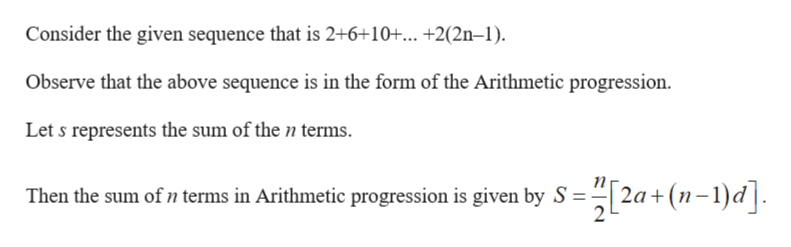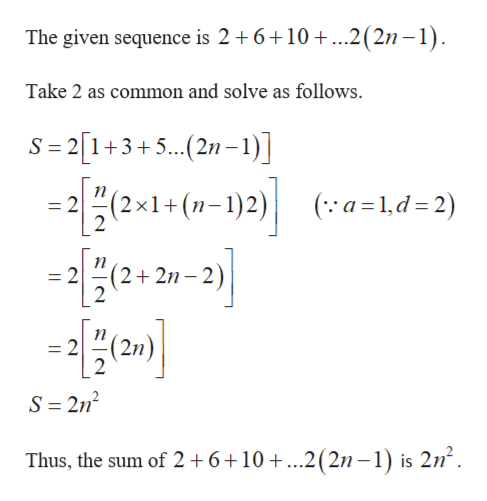1. Find a formula for the sum: 2+ 6+ 10 ... + 2(2n - 1)

Questionhelp_outlineImage Transcriptionclose1. Find a formula for the sum: 2+ 6+ 10 ... + 2(2n - 1) fullscreen
Step 1

The given sequence is 2+6+10+... +2(2n–1).

Step 2help_outlineImage TranscriptioncloseConsider the given sequence that is 2+6+10+... +2(2n-1) Observe that the above sequence is in the form of the Arithmetic progression Let s represents the sum of the n terms Then the sum of n terms in Arithmetic progression is given by S 2a( 2 L d fullscreen
Step 3help_outlineImage TranscriptioncloseThe given sequence is 2 6 10..2(2n -1) Take 2 as common and solve as follows. S 2[1+3+5.. (2n-1)] n = 2 2 (2x1+(-1)2) (a 1,d= 2) = 2(2+ 2n 21-2) n = 2 2 (2n S 2n2 Thus, the sum of 2 +6+10 +...2(2n-1) is 2n2 fullscreen

Want to see the full answer?

See Solution

Want to see this answer and more?

Our solutions are written by experts, many with advanced degrees, and available 24/7

See Solution
Tagged in

Math